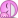# Intermediate Math Challenge - September 2018

• Challenge
• Featured
Mentor
Pardon the offtopic.
I find the introduction in the first post a bit strange. Summer is long gone nowOr you could be talking about summer 2019 or the folks that live their lives upside down.
Indian SummerLet $(X,d)$ be a metric space. Denote $*U = \mbox{Int}(U^c)$, where $U\subseteq X$ is open. Then $***U = *U$.
Proof. We have
$$***U = \mbox{Int}\left ( \mbox{Int} \left ( \mbox{Int}(U^c)\right )^c\right )^c$$
It holds that $(\mbox{Int}V)^c = \mbox{Cl}(V^c)$. Thus we have
$$\mbox{Int}\left ( \mbox{Int} \left ( \mbox{Int}(U^c)\right )^c\right )^c = \mbox{Int} \left (\mbox{ClCl}(U^c)\right ) = \mbox{Int}(U^c),$$
since the closure map is idempotent and $U^c$ is closed.
A work in progress. I've worked out that
$$U^c \subseteq \mbox{Int}(U^c) \Rightarrow (\mbox{Int}(U^c))^c \subseteq U\Rightarrow \mbox{Int}\left (\mbox{Int}(U^c)\right )^c\subseteq U$$
(inclusion in the other direction always holds) So I think it suffices to find a metrisable topology (not normable?) with an open set whose complement is not open. I tried messing around in $\mathbb R$ with the usual topology, but it's normable, I think that's too strong. Probably some embarrasingly trivial example. I am too tired now :(

Last edited:
Infrared
Gold Member
Let $(X,d)$ be a metric space. Denote $*U = \mbox{Int}(U^c)$, where $U\subseteq X$ is open. Then $***U = *U$.
Proof. We have
$$***U = \mbox{Int}\left ( \mbox{Int} \left ( \mbox{Int}(U^c)\right )^c\right )^c$$
It holds that $(\mbox{Int}V)^c = \mbox{Cl}(V^c)$. Thus we have
$$\mbox{Int}\left ( \mbox{Int} \left ( \mbox{Int}(U^c)\right )^c\right )^c = \mbox{Int} \left (\mbox{ClCl}(U^c)\right ) = \mbox{Int}(U^c),$$
since the closure map is idempotent and $U^c$ is closed.
Good, this is correct.
A work in progress. I've worked out that
$$U^c \subseteq \mbox{Int}(U^c) \Rightarrow (\mbox{Int}(U^c))^c \subseteq U\Rightarrow \mbox{Int}\left (\mbox{Int}(U^c)\right )^c\subseteq U$$
So I think it suffices to find a metrisable topology (not normable?) with an open set whose complement is not open. I tried messing around in $\mathbb R$ with the usual topology, but it's normable, I think that's too strong. Probably some embarrasingly trivial example. I am too tired now :(
Keep looking. Simple counterexamples exist.

Last edited:
Graah, I said it is probably something embarrasingly trivial
Take $\mathbb R$ with the standard topology. Then $U := \mathbb R\setminus\{0\}$ is open and $**U = \mathbb R$.
The previous proof contains calculation errors, fortunately the result doesn't change. For the sake of clarity I will (hopefully) give a better proof.
We use the identity $(\mbox{Int}V)^c = \mbox{Cl}(V^c)$
Firstly, take $V = (\mbox{Int}(U^c))^c$, then
$$***U = \mbox{Int} (\mbox{Int}V)^c = \mbox{Int}\mbox{Cl}(V^c) = \mbox{Int}\mbox{Cl}(\mbox{Int}(U^c)) = \mbox{Int}\mbox{Cl}\mbox{Int}(U^c).$$
Due to closedness $U^c = \mbox{Cl}(U^c)$. The composition map $\mbox{Int}\mbox{Cl}$ is idempotent thus we have
$$***U = \mbox{Int}\mbox{Cl}\mbox{Int}(U^c) = \mbox{Int}\mbox{Cl}\mbox{Int}\mbox{Cl}(U^c) = \mbox{Int}\mbox{Cl}(U^c) = \mbox{Int}(U^c) = *U.$$

The $***U = *U$ identity for open sets is more general. In fact, I didn't use any kind of specific topological property (such as the space being Hausdorff or even metrisable) to prove it. Hmm.

I'm interested in the motivation of the map ##*## (other than it being a more compact way of writing interior of complement). Is it used in development of theory somewhere?

Last edited:
•Infrared